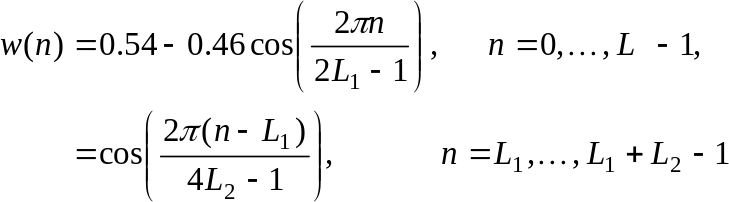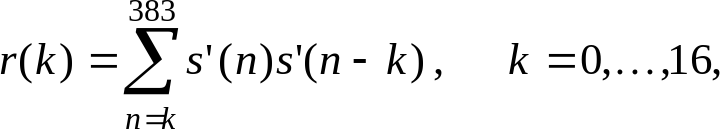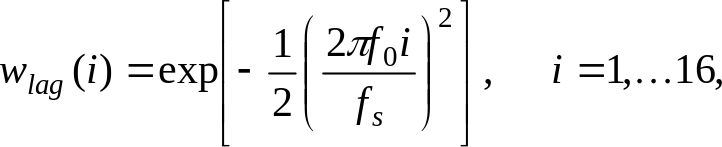## 5.2.1 Windowing and auto‑correlation computation

26.1903GPPAdaptive Multi-Rate - Wideband (AMR-WB) speech codecSpeech codec speech processing functionsTranscoding functionsTS

LP analysis is performed once per frame using an asymmetric window. The window has its weight concentrated at the fourth subframe and it consists of two parts: the first part is a half of a Hamming window and the second part is a quarter of a Hamming-cosine function cycle. The window is given by:( 6 )

where the values L1=256 and L2=128 are used.

The autocorrelations of the windowed speech s'(n),n=0,…,383 are computed by( 7 )

and a 60 Hz bandwidth expansion is used by lag windowing the autocorrelations using the window( 8 )

where f0=60 Hz is the bandwidth expansion and fs=12800 Hz is the sampling frequency. Further, r(0) is multiplied by the white noise correction factor 1.0001 which is equivalent to adding a noise floor at -40 dB.